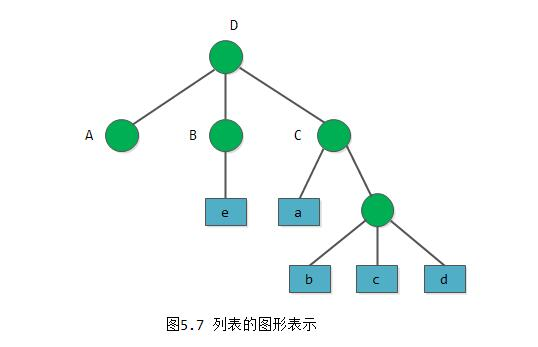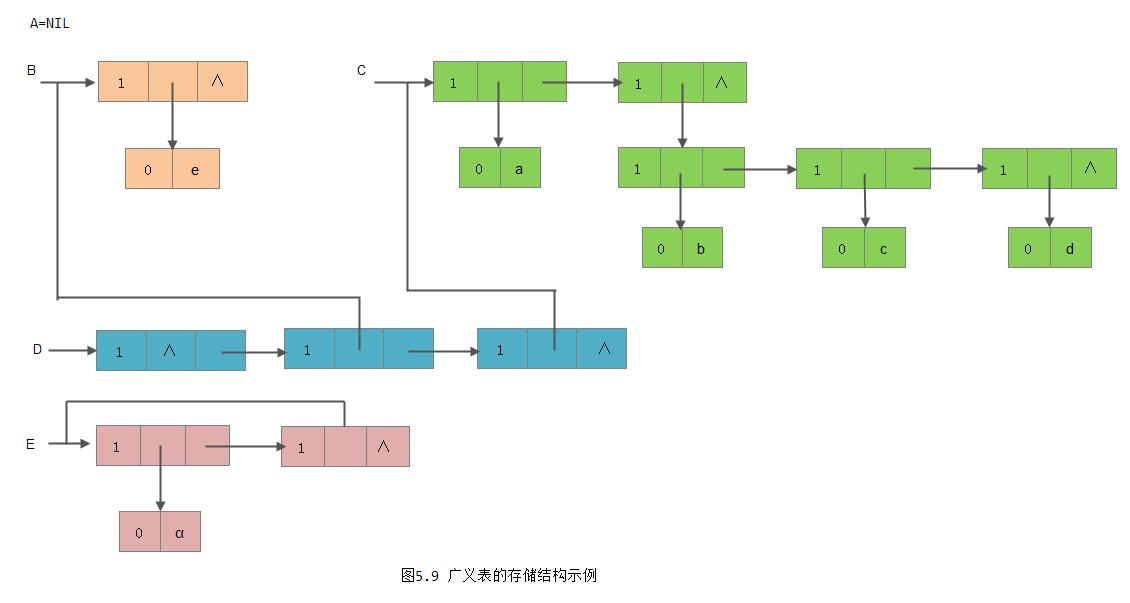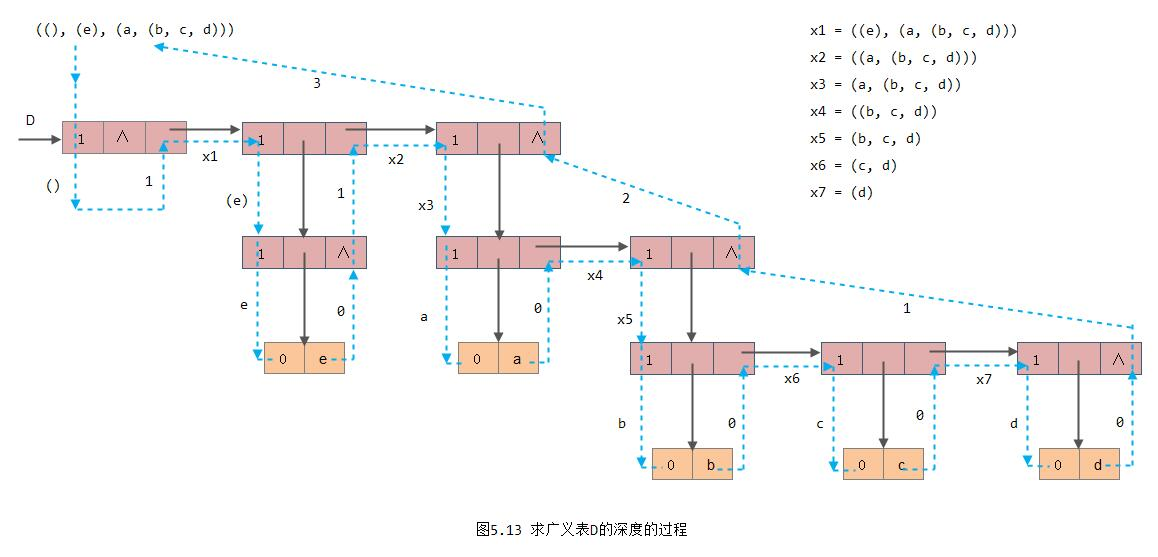## 1. 广义表的定义

(1) A = ()  -------  A是一个空表，它的长度为零

(2) B = (e) -------  列表B只有一个原子e，B的长度为1

(3) C = (a, (b, c, d)) -------  列表C的长度为2，两个元素分别为原子a和子表(b,c,d)

(4) D = (A, B, C) --------  列表D的长度为3， 其3个元素都是列表。显然，将子表的值代入后，择优D=((),(e), (a,(b,c,d)))

(5) E = (α, E) -------  这是一个递归的表，它的长度为2。 E相当于一个无限的列表E=(α, (α, (α, ...)))


1） 列表的元素可以是子表，而子表的元素还可以是子表……由此，列表是一个多层次的结构，可以用图形象地表示。例如图5.7表示的列表D。图中以圆圈表示列表，以方块表示原子。2) 列表可为其他列表所共享。例如，在上述例子中，列表A、B、C为D的子表，则在D中可以不必列出子表的值，而是通过子表的名称来引用。

3) 列表可以是一个递归的表，即列表也可以是其本身的一个子表。例如，列表E就是一个递归的表。

GetHead(B) = e,    GetTail(B) = ()

GetHead(D) = A,    GetTail(D) = (B, C)


GetHead((B, C)) = B, GetTail((B, C)) = (C)


## 2. 广义表的存储结构1） 除空表的表头指针为空外，对任何非空列表，其表头指针均指向一个表结点，且该结点中的hp域指示列表表头（或为原子结点，或为表结点)，tp域指向列表表尾（除非表尾为空，则指针为空，否则必为表结点）；

2）容易分清列表中原子和子表所在层次。如在列表D中，原子a和e在同一层次上，而b、c和d在同一层次且比a和e低一层， B和C是同一层的子表;

3) 最高层的表节点个数即为列表的长度。## 3. m元多项式的表示P = z((A,2), (B, 1), (15, 0))                  (5-7)

A = y((C,3), (D,2))
C = x((1, 10), (2,6))
D = x((3,5))

B = y((E,4), (F,1))
E = x((1,4), (6,3))
F = x((2,0))## 4. 广义表的递归算法

（1） 将编号为1至n-1的n-1个圆盘从X塔座移至Y塔座；

（2） 将编号为n的圆盘从X塔座移至Z塔座；

（3） 将编号为1至n-1的圆盘从Y塔座移至Z塔座。


### 4.1 求广义表的深度

     LS = (α1, α2, ..., αn)


      LS = (α1, α2, ..., αn)


算法5.5### 4.2 复制广义表

算法5.6

### 4.3 建立广义表的存储结构

(1) S = '()' (带括弧的空白串);

(2) S = (α1, α2, ..., αn)，其中αi(i=1,2,...,n)是S的子串


基本项：  置空广义表               当S为空表串时
建原子结点的子表          当S为单字符串时

对每一个si建立一个表节点，并令其hp域的指针为由si建立的子表的头指针，除最后建立的表结点的
尾指针为NULL外，其余表结点的尾指针均指向在它之后建立的表结点。


算法5.7

算法5.8

[参看]: